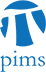## Discrete Math Seminar: Rational Distances with Rational Angles

• Date: 03/23/2010
Lecturer(s):

Frank De Zeeuw (UBC)

Ryan Schwartz (UBC)

Location:

University of British Columbia

Description:

Given n points in the real plane, the unit distance problem asks for
an asymptotic upper bound on the number of unit distances between
pairs of the points. We consider this problem under the restriction
that the line segments between the points make a rational angle (in
degrees) with the x-axis. In the complex plane, that allows us to
think of such segments of length 1 as roots of unity. Given a point
set with many such segments, we deduce simple linear equations with
many solutions in roots of unity. Using an algebraic theorem of Mann
from 1965, we can give a uniform bound on the number of such
solutions, which will give us a tight asymptotic bound on the number
of unit distances with rational angles. These results can then be
extended to rational distances. This is joint work with Jozsef
Solymosi.

Schedule:

4:00 - 5:00pm, WMAX 216.